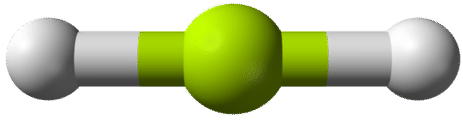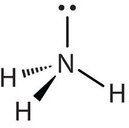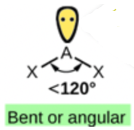4.7: The Shapes of Molecules

Learning Objectives

• Predict the general shape of a simple covalent molecule.

Molecular Shape: VSEPR Theory

Unlike ionic compounds, with their extended crystal lattices, covalent molecules are discrete units with specific three-dimensional shapes. The shape of a molecule is determined by the fact that covalent bonds, which are composed of negatively charged electrons, tend to repel one another. This concept is called the valence shell electron pair repulsion (VSEPR) theory. For example, the two covalent bonds in $$\ce{BeCl2}$$ stay as far from each other as possible, ending up 180° apart from each other. The result is a linear molecule:The three covalent bonds in BF3 repel each other to form 120° angles in a plane, in a shape called trigonal planar:The molecules $$\ce{BeCl2}$$ and $$\ce{BF3}$$ actually violate the octet rule; however, such exceptions are rare and will not be discussed in this text.

Try sticking three toothpicks into a marshmallow or a gumdrop and see if you can find different positions where your “bonds” are farther apart than the planar 120° orientation.

The four covalent bonds in CCl4 arrange themselves three dimensionally, pointing toward the corner of a tetrahedron and making bond angles of 109.5°. CCl4 is said to have a tetrahedral shape:Atoms Around Central Atom Geometry Example
2 $$\ce{AB_2}$$ Linear $$\ce{BeCl_2}$$
3 $$\ce{AB_3}$$ Trigonal Planar $$\ce{BF_3}$$
4 $$\ce{AB_4}$$ Tetrahedral $$\ce{CCl_4}$$

In determining the shapes of molecules, it is useful to first determine the Lewis diagram for a molecule. The shapes of molecules with multiple bonds are determined by treating the multiple bonds as one bond. Thus, formaldehyde (CH2O) has a shape similar to that of BF3. It is trigonal planar.Molecules With Lone Pairs Around Central Atom

Molecules with lone electron pairs around the central atom have a shape based on the position of the atoms, not the electron pairs. For example, NH3 has one lone electron pair and three bonded electron pairs. These four electron pairs repel each other and adopt a tetrahedral arrangement. However, the shape of the molecule is described in terms of the positions of the atoms, not the lone electron pairs. Thus, NH3 is said to have a trigonal pyramidal shape, not a tetrahedral one.Similarly, H2O has two lone pairs of electrons around the central oxygen atom and two bonded electron pairs. Although the four electron pairs adopt a tetrahedral arrangement, the shape of the molecule is described by the positions of the atoms only. The shape of H2O is bent with an approximate 109.5° angle.In summary, to determine the molecular geometry:

Step 1: Draw the Lewis structure.

Step 2: Count the number of bonds (a double/triple bond counts as one) and lone pairs around the central atom.

Step 3: Use Table 4.5.1 to determine the molecular geometry.Table 4.5.1: The molecular geometry depends on the number of bonds and lone pairs around the central atom, A.

Example $$\PageIndex{1}$$

What is the geometry of the ammonium ion, NH4+? Its Lewis structure is shown below. How is this different from ammonia, NH3?Solution

In ammonium ion, the central atom N has 4 bonds and no lone pair. It is equivalent to the below in Table 4.5.1. Hence, this is tetrahedral.In ammonia (NH3), shown below, N has 3 bonds and one lone pair.It is equivalent to the below in Table 4.5.1. Hence, the shape of this molecule is trigonal pyramid.Exercise $$\PageIndex{1}$$

What is the molecular shape of nitrosyl chloride, a highly corrosive, reddish-orange gas? Its Lewis structure is shown below.